# Mark III Systems Blog

##### Hyperparameters for Classifying Images with Convolutional Neural Networks – Part 2 – Batch Size

In this two part series, I discuss what I consider to be two of the most important hyperparameters that are set when training convolutional neural networks (CNNs) for image classification or object detection.  These are learning rate and batch size.  In this second part, I will discuss batch size.  For an overview of CNNs, please see part 1 of this series.

What is batch size and why is it important?

First, a little background on neural networks.  When training a neural network, weights and biases are used to determine the importance of each value in the input. For example, if the input is a 768x1 vector of values, the network needs to figure out the relative importance of each of these separate values as it pertains to the performance of the network.  This is largely dependent on the weights between neurons.  During training, an input value is multiplied by a weight (and a bias potentially added).  The resulting value is passed to the next neuron where the calculated value is the input for an activation function.  See the image below for a visual explanation of the math.  The important thing to know is that each of these weights is often initially set to random values.  When the input values are passed through the network during the forward pass, a loss value is calculated at the end.  That loss value is then used during a process known as back propagation.  During back prop, the weights are updated in an effort to make the network more accurate in its predictions.  How often back propagation occurs is dependent upon batch size.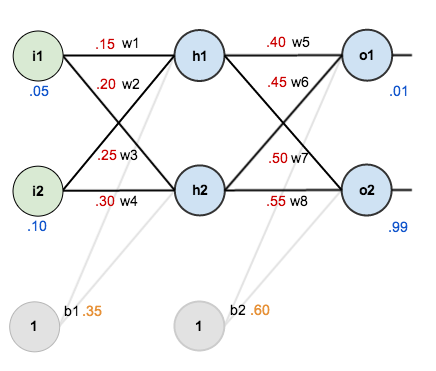Image from here.

If the batch size is set to 1, then every time an input passes through the network, back prop will occur and weights will be updated.  This can be good because updates are occurring often and can potentially make the performance of the network very good due to how often the weights are updated.  This can be bad, though, because the weights are being updated after every input passes through the network.  Imagine a network that has millions of weights (Inception-v3 has 24 million parameters).  Each time back prop occurs, all of these weights have to be updated.  Now imagine that happening hundreds of thousands, or even millions of times as inputs are passed through the network.  This is very computationally intensive and time consuming.  Low batch sizes can produce very accurate results, but at the cost of computing power and time.

One way to decrease training time and computational power, is to increase the batch size to say 16, 32, 256, 1024,or higher.  When the batch size is greater than 1, something interesting occurs.  A number of inputs equal to the batch size are passed through the network.  The calculated loss for each of these inputs is then averaged and that value is used to update the weights.  So if the batch size is 32, then 32 inputs are passed through the network before back prop occurs.  What are the pros and cons of this?  With less back prop occurring, computational requirements decrease and training time decreases.  However, the loss value is now an average of multiple inputs rather than just the value derived from a single input.  Thus, the weights are updated based on an average of several inputs.  This can cause the weight updates to be less granular, resulting in an overall decrease in accuracy and potentially a lack of ability to generalize to new data.

Comparing the effects of different batch sizes on a dataset

Here are the results from some image classification experiments I conducted using Nvidia's DIGITS software running on an Nvidia DGX-1.  I used the same input images as in Part 1 and the learning rate was the same for each experiment.  75% of the images were used for training, 25% were used for validation.  The difference in these experiments was that the batch size was changed.

In each Tensorboard image below, as in Part 1, the two things to look at are the training loss values in blue and training accuracy values in red. Yes, I know there is more to it than that when evaluating how a model is performing, but for illustration, this is enough. The batch sizes were 1, 2, 4, and 8, and the base learning rate was 0.00001.  We want to see the loss go down and the accuracy to go up. ​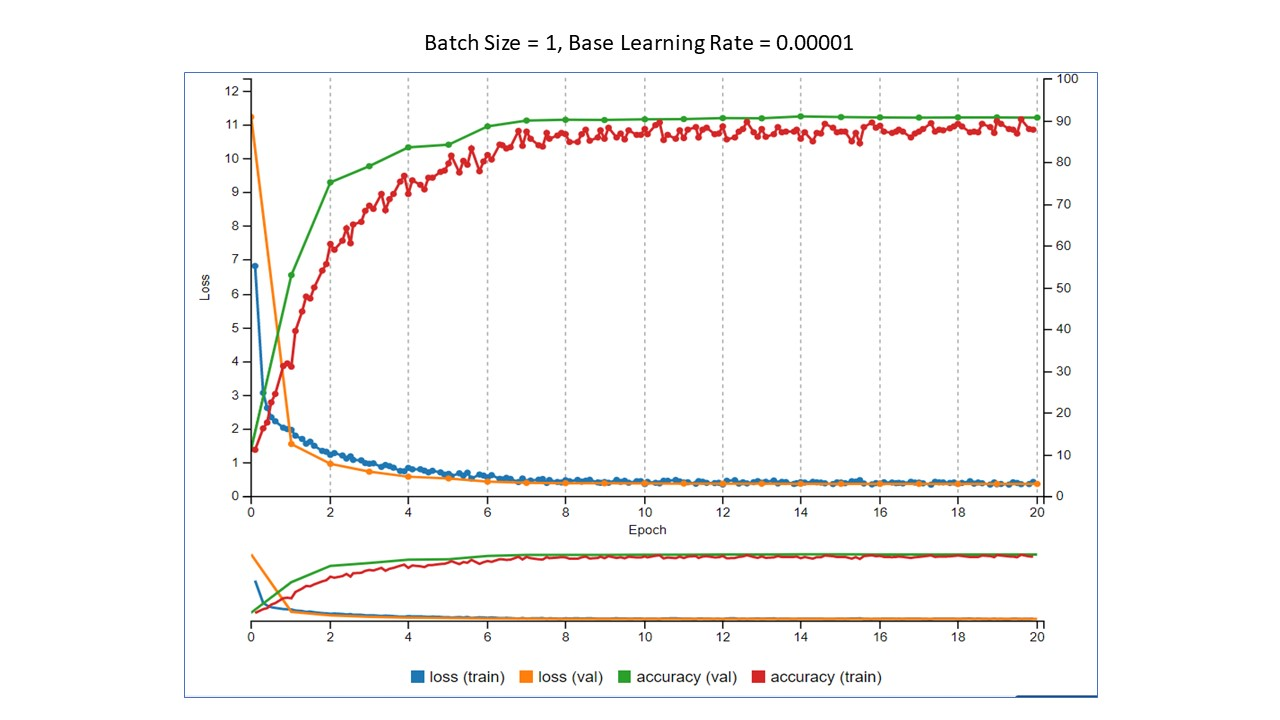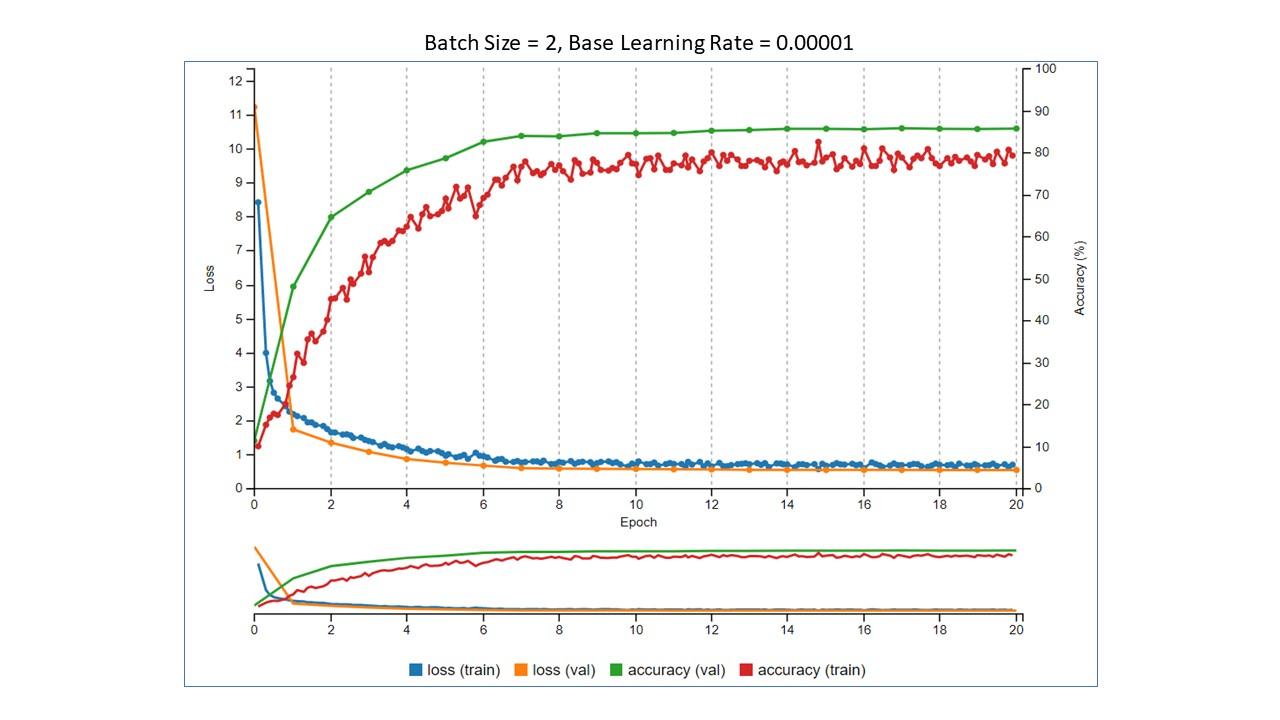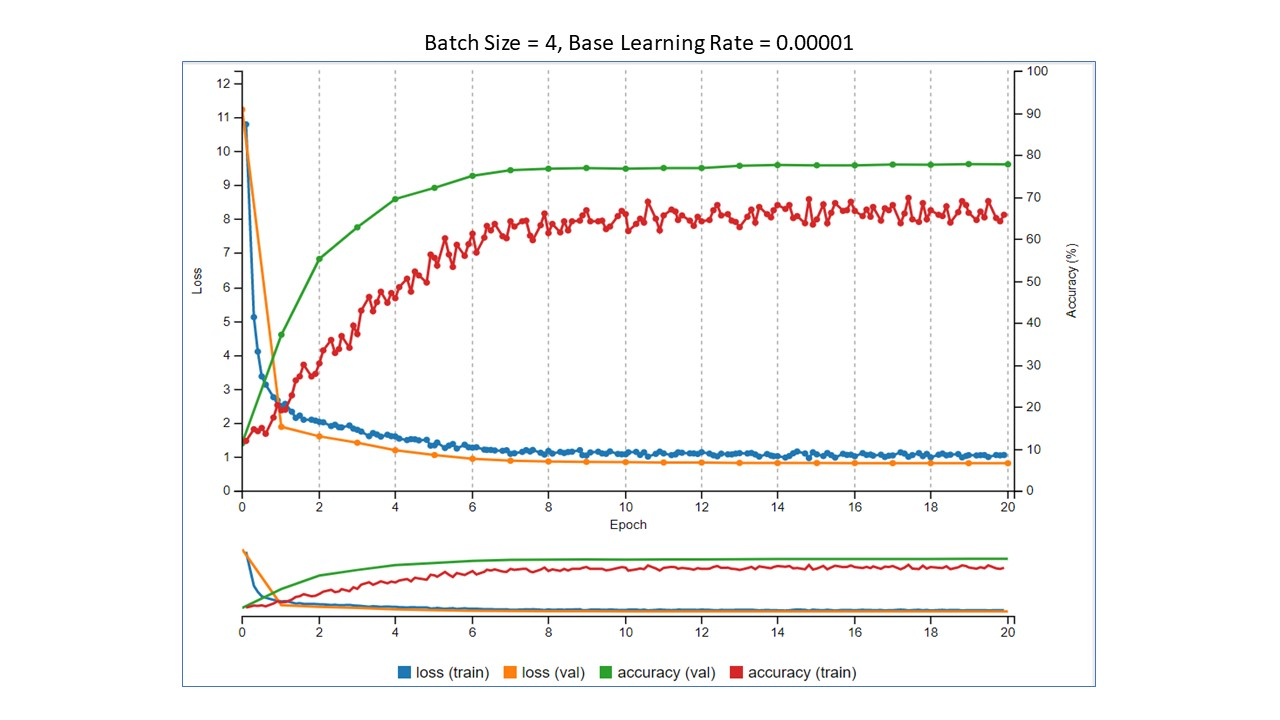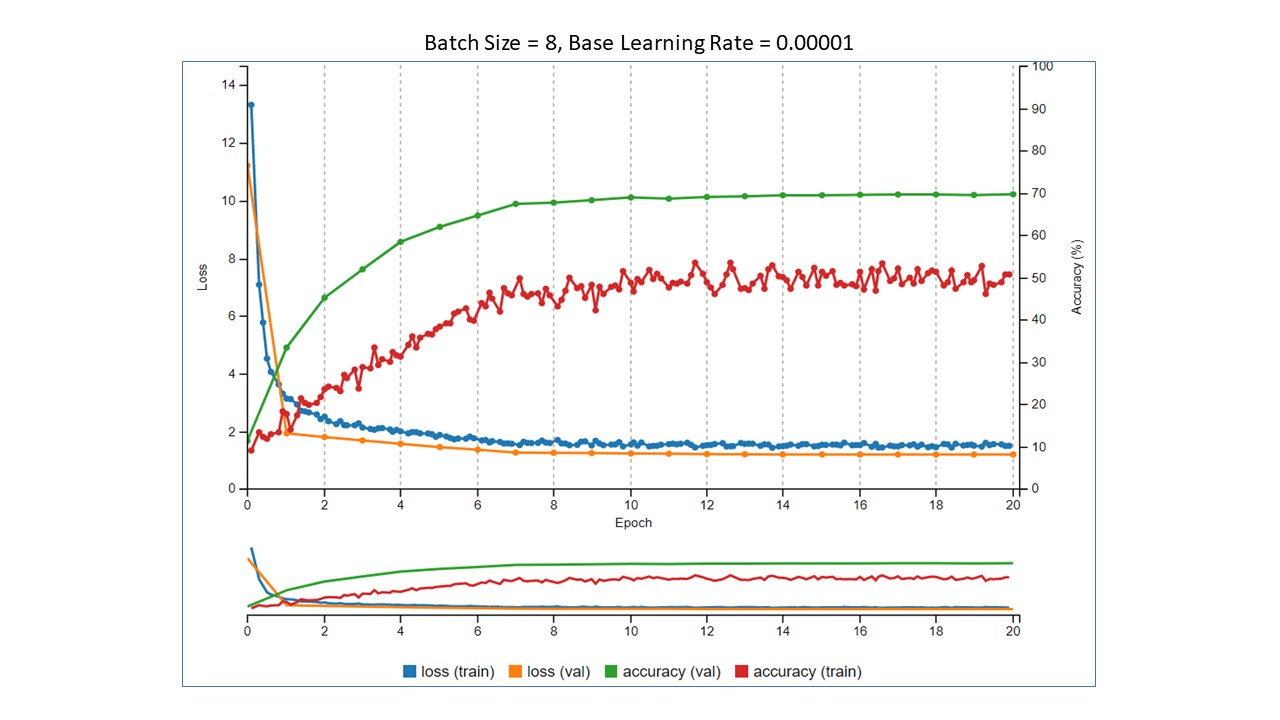Summary of the results

• As the batch size increased, the accuracy decreased.
• As the batch size increased, the running time also decreased.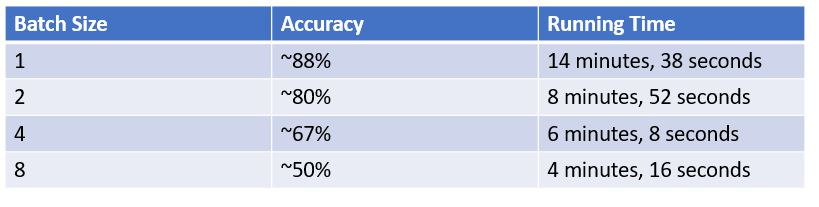The question now becomes, "Which is more important?  Accuracy or training time?"  With this very small data set and small number of epochs, the training did not take terribly long.  However, imagine that your training data set is 500,000 images and takes 12 hours to train.  In this small experiment, by simply changing the batch size from 1 to 2, we were able to decrease the training time by almost 40%.  In your scenario, that would decrease the training time from 12 hours to 7 hours 12 minutes.  That is a significant decrease.  The decrease in training time is not without a trade off, though.  Training time decreased, but so did accuracy.  If you need high accuracy, then decreasing the batch size may be a problem.  If 80% accuracy is acceptable to have a 40% reduction in training time, then simply changing the batch size can do that for you.  One thing to remember, though, is that these values are derived from training on a small data set for a small number of epochs.  Your results will definitely vary, but will should be similar.  That's where the fun part of experimenting comes in!